Anzeige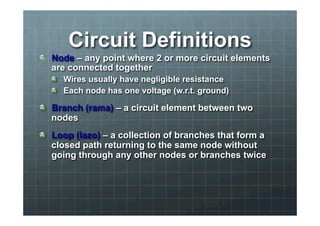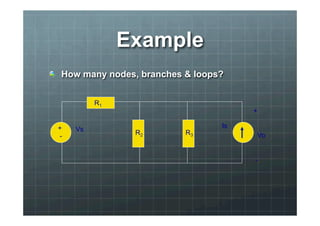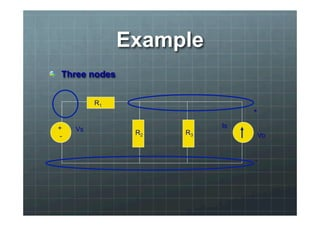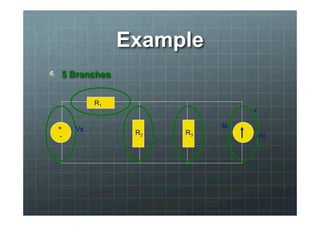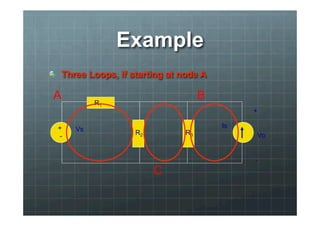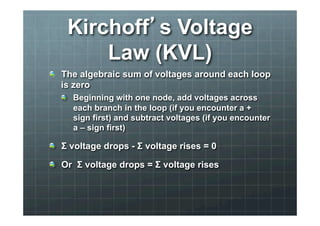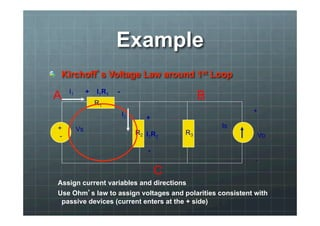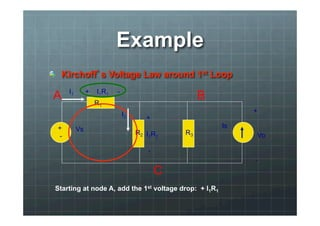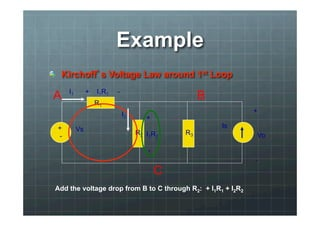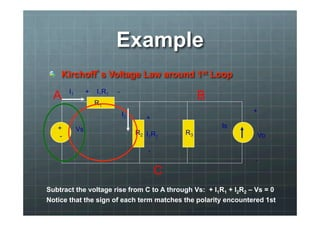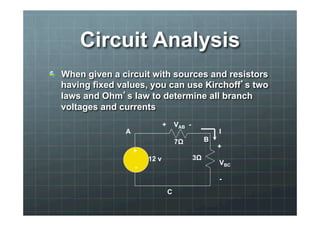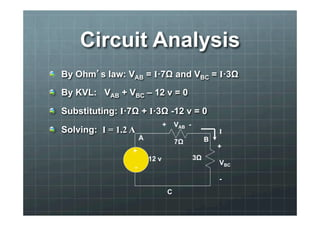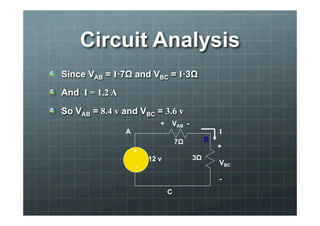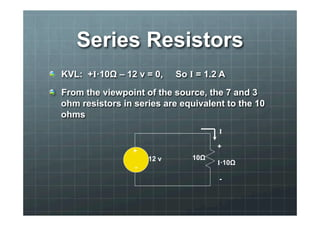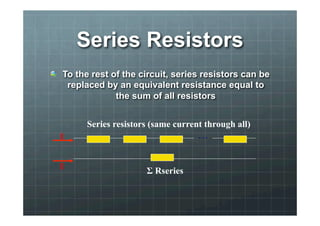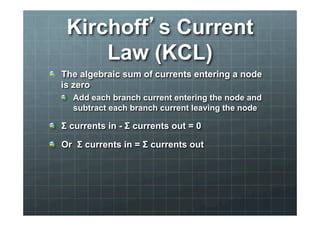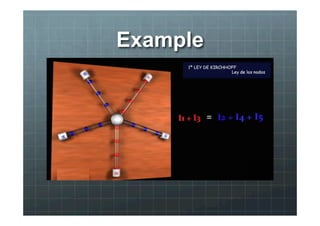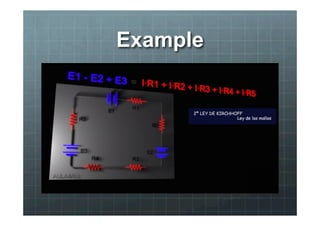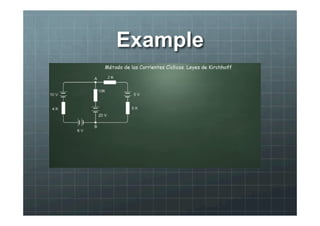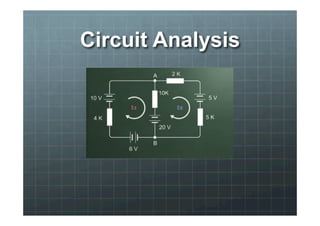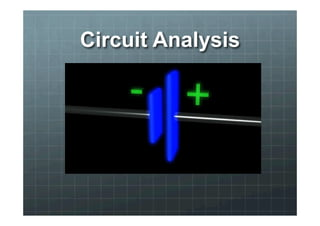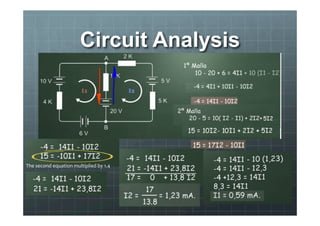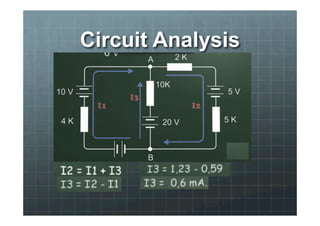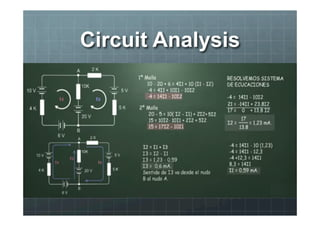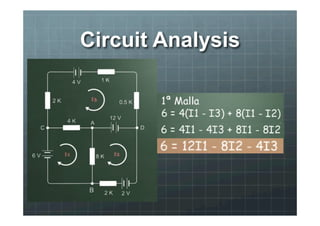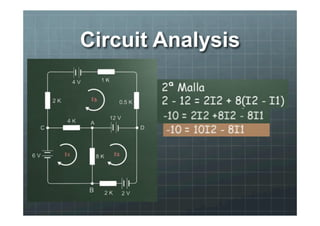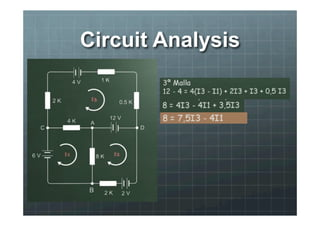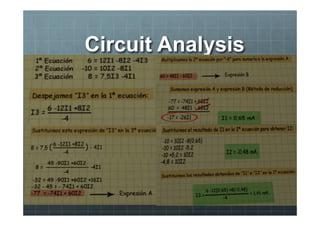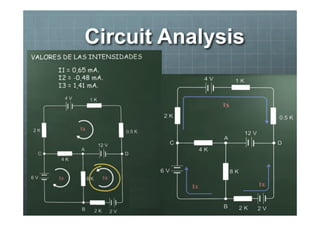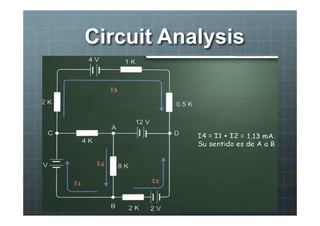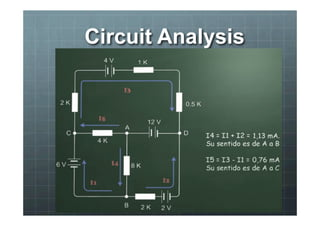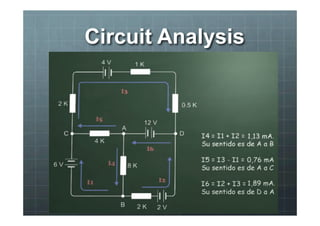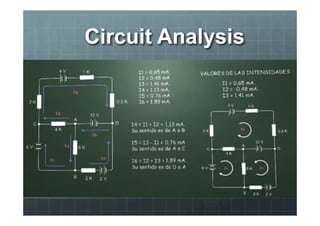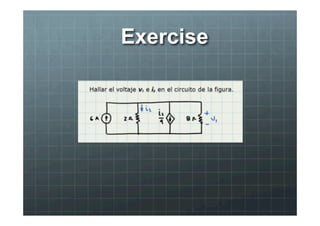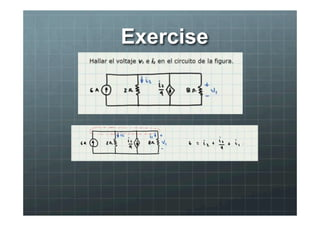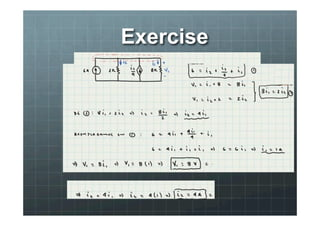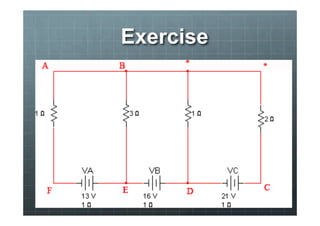1 von 37
Anzeige

### Kirchoff

1. Circuit Definitions ! Node – any point where 2 or more circuit elements are connected together ! Wires usually have negligible resistance ! Each node has one voltage (w.r.t. ground) ! Branch (rama) – a circuit element between two nodes ! Loop (lazo) – a collection of branches that form a closed path returning to the same node without going through any other nodes or branches twice
2. Example ! How many nodes, branches & loops? + - R1 Vs Is R2 R3 + Vo -
3. Example ! Three nodes + - R1 Vs Is R2 R3 + Vo -
4. Example ! 5 Branches + - R1 Vs Is R2 R3 + Vo -
5. Example ! Three Loops, if starting at node A + - R1 Vs Is R2 R3 + Vo - A B C
6. Kirchoff’s Voltage Law (KVL) ! The algebraic sum of voltages around each loop is zero ! Beginning with one node, add voltages across each branch in the loop (if you encounter a + sign first) and subtract voltages (if you encounter a – sign first) ! Σ voltage drops - Σ voltage rises = 0 ! Or Σ voltage drops = Σ voltage rises
7. Example ! Kirchoff’s Voltage Law around 1st Loop + - R1 Vs Is R2 R3 + Vo - A B C I2 I1 + I2R2 - + I1R1 - Assign current variables and directions Use Ohm’s law to assign voltages and polarities consistent with passive devices (current enters at the + side)
8. Example ! Kirchoff’s Voltage Law around 1st Loop + - R1 Vs Is R2 R3 + Vo - A B C I2 I1 + I2R2 - + I1R1 - Starting at node A, add the 1st voltage drop: + I1R1
9. Example ! Kirchoff’s Voltage Law around 1st Loop + - R1 Vs Is R2 R3 + Vo - A B C I2 I1 + I2R2 - + I1R1 - Add the voltage drop from B to C through R2: + I1R1 + I2R2
10. Example ! Kirchoff’s Voltage Law around 1st Loop + - R1 Vs Is R2 R3 + Vo - A B C I2 I1 + I2R2 - + I1R1 - Subtract the voltage rise from C to A through Vs: + I1R1 + I2R2 – Vs = 0 Notice that the sign of each term matches the polarity encountered 1st
11. Circuit Analysis ! When given a circuit with sources and resistors having fixed values, you can use Kirchoff’s two laws and Ohm’s law to determine all branch voltages and currents + 12 v - I + VAB - 7Ω 3Ω A B C + VBC -
12. Circuit Analysis ! By Ohm’s law: VAB = I·7Ω and VBC = I·3Ω ! By KVL: VAB + VBC – 12 v = 0 ! Substituting: I·7Ω + I·3Ω -12 v = 0 ! Solving: I = 1.2 A + + VAB - A B 12 v - I 7Ω 3Ω C + VBC -
13. Circuit Analysis ! Since VAB = I·7Ω and VBC = I·3Ω ! And I = 1.2 A ! So VAB = 8.4 v and VBC = 3.6 v + + VAB - 12 v - I 7Ω 3Ω A B C + VBC -
14. Series Resistors ! KVL: +I·10Ω – 12 v = 0, So I = 1.2 A ! From the viewpoint of the source, the 7 and 3 ohm resistors in series are equivalent to the 10 ohms + 12 v - I 10Ω + I·10Ω -
15. Series Resistors ! To the rest of the circuit, series resistors can be replaced by an equivalent resistance equal to the sum of all resistors Series resistors (same current through all) . . . Σ Rseries I I
16. Kirchoff’s Current Law (KCL) ! The algebraic sum of currents entering a node is zero ! Add each branch current entering the node and subtract each branch current leaving the node ! Σ currents in - Σ currents out = 0 ! Or Σ currents in = Σ currents out
17. Example ! Kirchoff’s Current Law at B
18. Example ! Kirchoff’s Current Law at B
19. Example ! Kirchoff’s Current Law at B
20. Circuit Analysis
21. Circuit Analysis
22. Circuit Analysis The second equation multiplied by 1.4
23. Circuit Analysis
24. Circuit Analysis
25. Circuit Analysis
26. Circuit Analysis
27. Circuit Analysis
28. Circuit Analysis
29. Circuit Analysis
30. Circuit Analysis
31. Circuit Analysis
32. Circuit Analysis
33. Circuit Analysis
34. Exercise
35. Exercise
36. Exercise
37. Exercise
Anzeige